# Darboux sum

A sum of special type. Let a real functionbe defined and bounded on a segment, let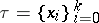be a decomposition of: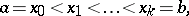and set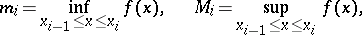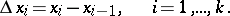The sums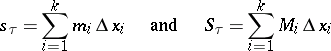are known, respectively, as the lower and upper Darboux sums. For any two decompositionsand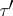ofthe inequality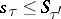is valid, i.e. any lower Darboux sum is no larger than an upper. Ifis a Riemann sum, then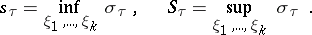The geometric meaning of the lower and upper Darboux sums is that they are equal to the planar areas of stepped figures consisting of rectangles whose base widths are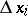and with respective heights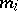and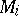(see Fig.) if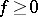. These figures approximate, from the inside and outside, the curvilinear trapezium formed by the graph of, the abscissa axis and the rectilinear segments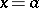and(which may degenerate into points).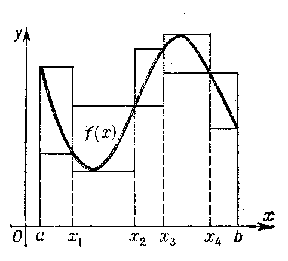Figure: d030160a

The numbers(1)

are called, respectively, the lower and the upper Darboux integrals of. They are the limits of the lower and the upper Darboux sums: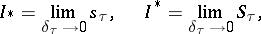where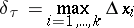is the fineness (mesh) of the decomposition. The condition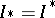(2)

is necessary and sufficient for a functionto be Riemann integrable on the segment. Here, if condition (2) is met, the value of the lower and the upper Darboux integrals becomes identical with the Riemann integral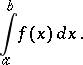With the aid of Darboux sums, condition (2) may be formulated in the following equivalent form: For eachthere exists a decompositionsuch thatThe condition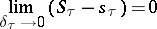is also necessary and sufficient for the Riemann integrability ofon. Herewhereis the oscillation (cf. Oscillation of a function) ofonThe concept of lower and upper Darboux sums may be generalized to the case of functions of several variables which are measurable in the sense of some positive measure. Letbe a measurable (for example, Jordan or Lebesgue) subset of the-dimensional space,and suppose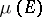is finite. Letbe a decomposition of, i.e. a system of measurable subsets ofsuch that(3)(4)

Let a functionbe bounded onand let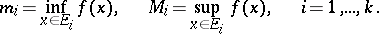(5)

The sums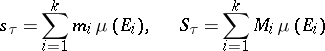(6)

are also said to be, respectively, lower and upper Darboux sums. The lowerand the upperintegrals are defined by formulas (1). For Jordan measure, their equality is a sufficient and necessary condition for the function to be Riemann integrable, and their common value coincides with the Riemann integral. For Lebesgue measure, on the other hand, the equality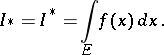is always valid for bounded Lebesgue-measurable functions.

In general, ifis a complete-additive bounded measure, defined on a-algebra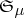, ifis a bounded measurable real-valued function on, if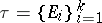is a decomposition of a set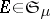into-measurable sets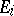which satisfy the conditions (3) and (4), and if the Darboux sumsand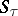are defined by formulas (5) and (6), while the integrals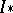and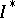are defined by the formulas (1), in whichis always understood to mean the measure under consideration, then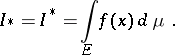A generalization of the Darboux sums to unbounded-measurable functionsdefined on setsare the series (if they are absolutely convergent)(7)

where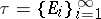is a decomposition of(this decomposition consists, generally speaking, of an infinite number of-measurable setswhich satisfy condition (4) and are, of course, such that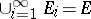), whileandare defined by (5). In (7) (as in (6) above) it is assumed that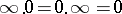. Ifandare again defined according to (1) and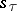andare now defined in the sense of (7) and exist for each, then. If the value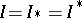is finite, thenis integrable with respect toand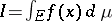.

Named after G. Darboux .